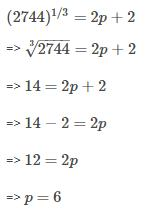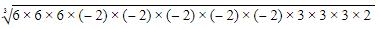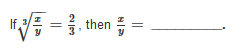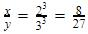Courses

# Test: Cubes And Cube Roots- 3

## 20 Questions MCQ Test Mathematics (Maths) Class 8 | Test: Cubes And Cube Roots- 3

Description
This mock test of Test: Cubes And Cube Roots- 3 for UPSC helps you for every UPSC entrance exam. This contains 20 Multiple Choice Questions for UPSC Test: Cubes And Cube Roots- 3 (mcq) to study with solutions a complete question bank. The solved questions answers in this Test: Cubes And Cube Roots- 3 quiz give you a good mix of easy questions and tough questions. UPSC students definitely take this Test: Cubes And Cube Roots- 3 exercise for a better result in the exam. You can find other Test: Cubes And Cube Roots- 3 extra questions, long questions & short questions for UPSC on EduRev as well by searching above.
QUESTION: 1

### A natural number is said to be a perfect cube, if it is the cube of some _________.

Solution:

The cube of a natural number is always a natural number.

QUESTION: 2

### The cube of 23 is ___________

Solution:

23= 23×23×23 = 12167

QUESTION: 3

### Find the cube of 75.

Solution:
QUESTION: 4

If n = m × m × m = m3, where m is an integer, then n is a perfect cube and the number m is called the __________ of n.

Solution:
QUESTION: 5

If (2744)1/3 = 2p+2, then the value of P is

Solution:QUESTION: 6

The cube root of 13824 is __________.

Solution:
By prime factorisation:
13824 is (2x2x2)x(2x2x2)x(2x2x2)x(3x3x3)
Making pairs of three three numbers
which gives 2x2x2x3=24
Therefore cube root of 13824 is 24
QUESTION: 7

The square of a natural number subtracts from its cube comes 100. The number is __________.

Solution:
QUESTION: 8

Find the cube root of 0.001331.

Solution:
QUESTION: 9

Find the smallest number by which 54 must be multiplied so that the product is a perfect cube.

Solution:
QUESTION: 10

Find the ones digit of cube root of 2197.

Solution:

2197

Unit's place of 2197 = 7

The ones digit of cube root of 2197 will be 3 as 3= 27 which has 7 as unit's place

QUESTION: 11

Find the cube root of -5832.

Solution:
QUESTION: 12

The cube root of the 216 x (−32) x 54 is ________

Solution:

We have 216 x (-32) x 54.
Applying prime factorisation giveswhich gives  -(6 x 2 x 2 x 3)= -72.

QUESTION: 13

If x is ones digit and y is tens digit of a two digit number, then the cube of the number will be _________.

Solution:
QUESTION: 14

If 72K is a perfect cube, find the value of K.

Solution:

72 = 2×2××2×3×3

If  K = 3, 72K = 72 × 3 = 216 = 63

is a perfect cube.

QUESTION: 15

If (504 + p) is a perfect cube number, whose cube root is p, then p = ______.

Solution:

Dear Student, we know that 83= 512
So p = 512−504 = 8

QUESTION: 16

23 is a cube root of _________.

Solution:
QUESTION: 17

Find the side of the cubical box whose volume is 474.552 dm3.

Solution:
QUESTION: 18

If volume of cube is 4913cm³ then length of side of cube is

Solution:
QUESTION: 19

Divide 5673375 by the smallest number so that the product is perfect cube.

Solution:
QUESTION: 20Solution:

Taking cube both the sides,